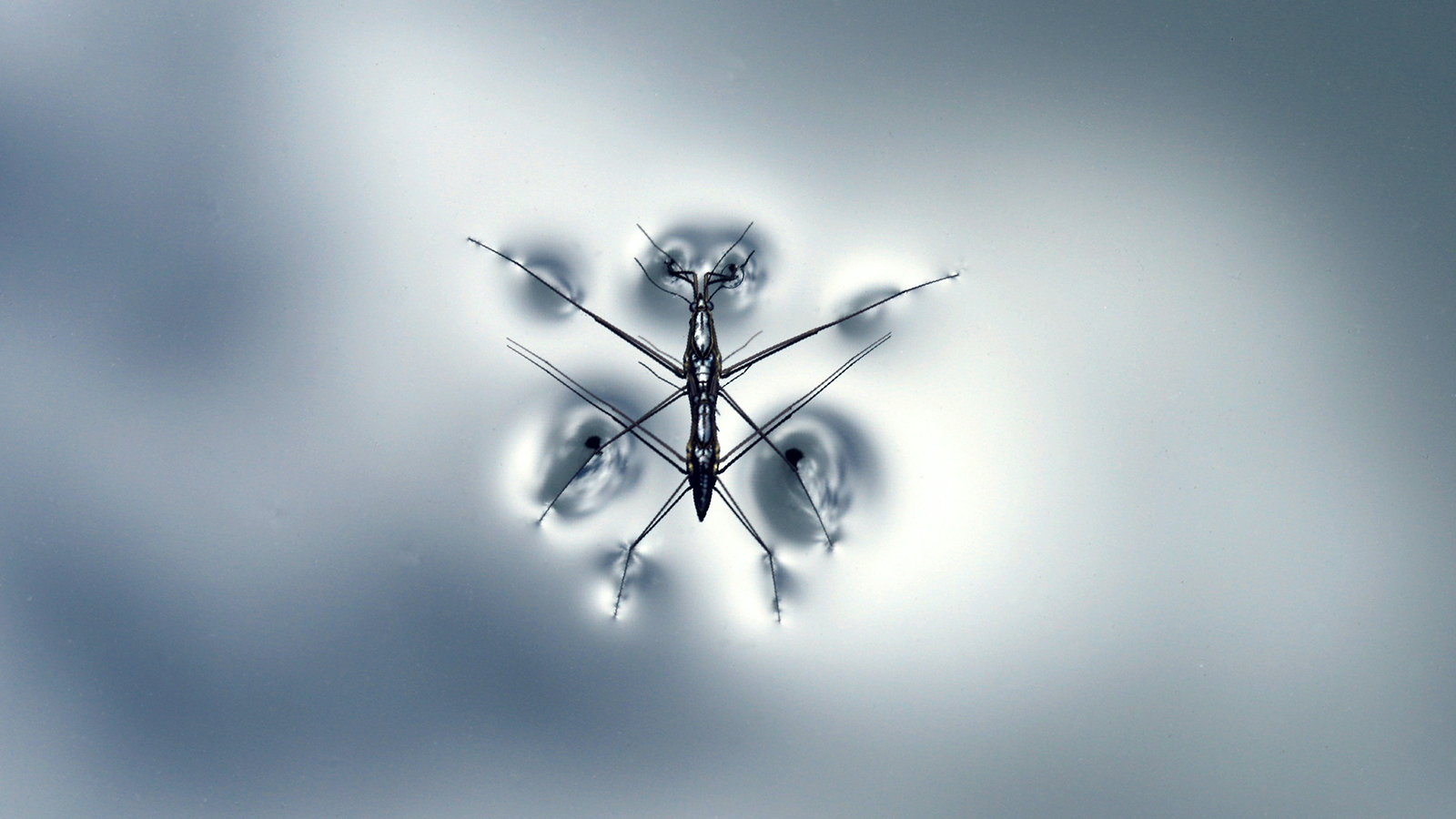# Surface Tension Measurement

Surface tension measurements are done using either force or optical tensiometers. Surface tension is an important parameter in many industrial processes which makes its measurement mandatory for process and product optimization.

## What is Surface Tension?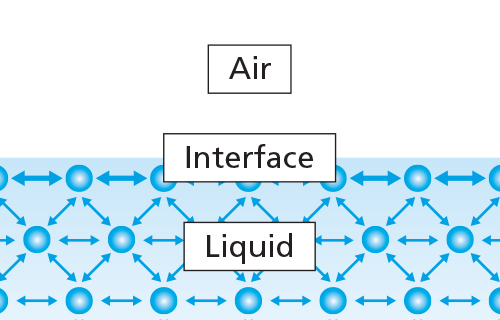Surface tension arises from cohesive forces between the liquid molecules. In the bulk, molecules are interacting equally with each other in all directions. However, at the surface, molecules don't have the same neighbors on all sides. Thus, a net inward force pulls the molecules toward the bulk. This gives rise to a property called surface tension. How high the surface tension is, is dependent on the type of molecular interactions present. For example, the surface tension of water is high, as the water molecules interact with each other through hydrogen bonds which are relatively strong.

There are several different units for surface and interfacial tension; typically mN/m (which is equivalent to dynes/cm) is used.

Surface tension measurements are widely used in many industrial areas as it has a direct effect on the spreading of the coating formulation and it's used to characterize surfactant solutions among others.## Surface Tension Measurement with Force Tensiometers

Measurement of surface tension can be performed with a force tensiometer. These instruments are based on measuring the forces exerted on a probe that is positioned at the liquid-gas or liquid-liquid interface. The probe is connected to a very sensitive balance and the liquid interface of interest is brought into contact with the probe. The forces measured by the balance as the probe interacts with the surface of the liquid can be used to calculate the surface tension. The force depends on the following factors: size and shape of the probe, contact angle between the probe and the liquid, and the surface tension of the liquid. The size and shape of the probe are easily controlled. Probes are typically made of platinum which helps to ensure a zero degree contact angle between the probe and the liquid to be studied. Two configurations of probes are commonly used; the du Noüy ring and the Wilhelmy plate. A metal rod can also be used instead of a Wilhelmy plate when sample volume is limited.

### Du Noüy ring method

This method utilizes a platinum ring as the probe. The ring is submerged below the interface by moving the stage where the liquid container is placed. After immersion, the stage height is gradually decreased and the ring eventually pulls through the interface bringing with it a meniscus of liquid. If the container is lowered further eventually the meniscus will tear from the ring. Before this event, the volume (and thus the force exerted) of the meniscus passes through the maximum value and begins to drop before the actual tearing event.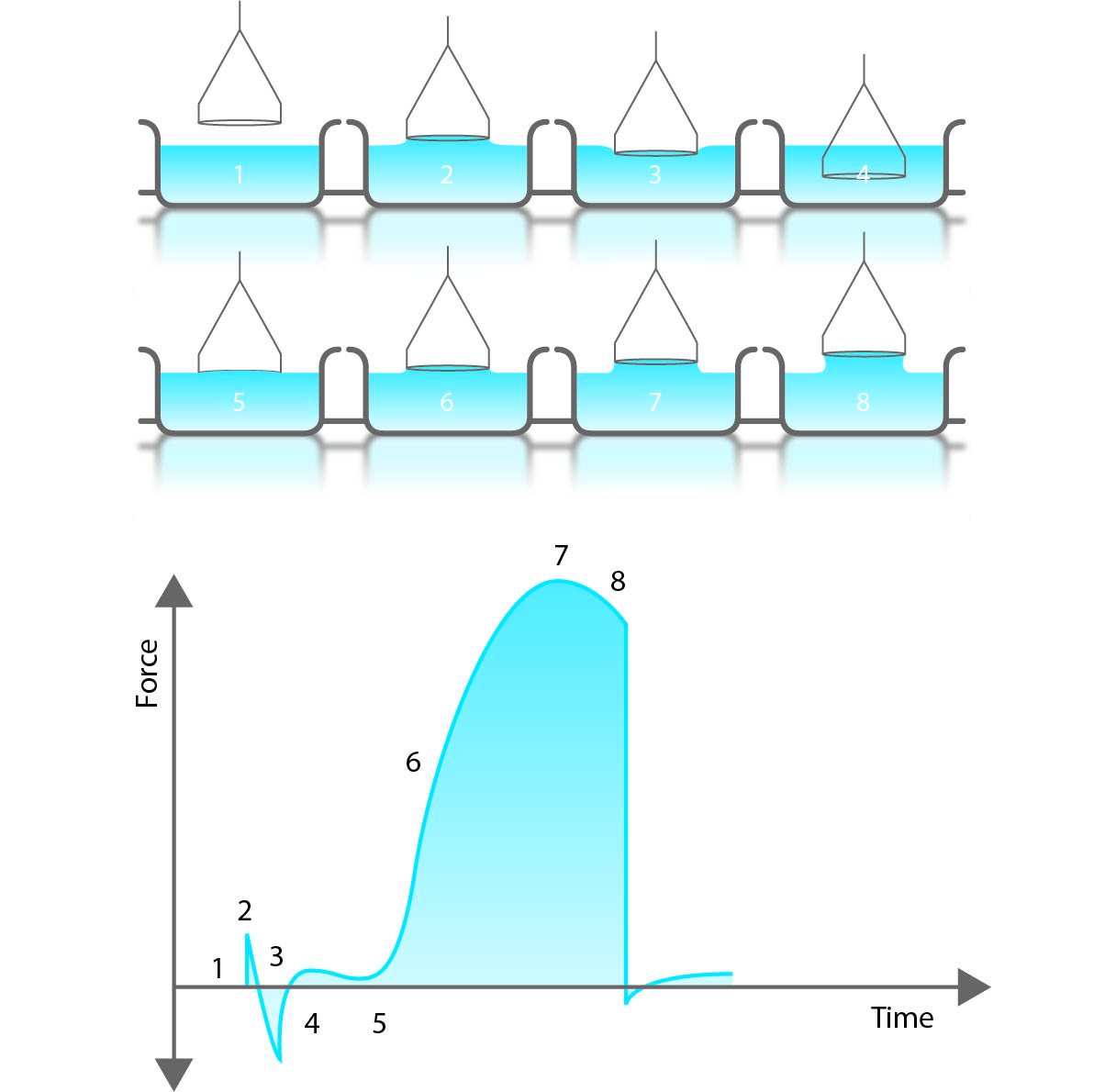The calculation of the surface tension is based on the measurement of the maximum force. The depth of immersion of the ring and the level to which the ring is raised when it experiences the maximum pull is irrelevant to this technique. Original calculations are based on a ring with an infinite diameter (or wire) and do not consider the excess liquid that is pulled up due to the proximity of one side of the ring to the other. The portion of the fluid which is directly under the ring is not there due to surface tension forces – but rather capillary forces. This liquid, however, contributes to a force reading given by the balance and would thus increase the measured surface tension value by approximately 7 %. Today correction factors are routinely used by automated software calculations to subtract and account for this excess liquid. When using a correction factor, the density of the liquid, or in case of interfacial tension measurement density difference between the two liquids, must be known.

### Wilhelmy plate method

This method utilizes a rough platinum plate as the probe. The calculations of this technique are based on the perimeter of the fully wetted plate in contact with the liquid. In this method the position of the probe relative to the surface is significant. As the liquid surface is brought into contact with the probe, the instrument will detect the exact moment of contact by registering a change in force on the probe. This position will thus be labeled the “zero depth of immersion”. The plate is then immersed to a set depth below this value (typically a few mm into the interface). When the plate is later returned to the zero-depth of immersion, the force is recorded.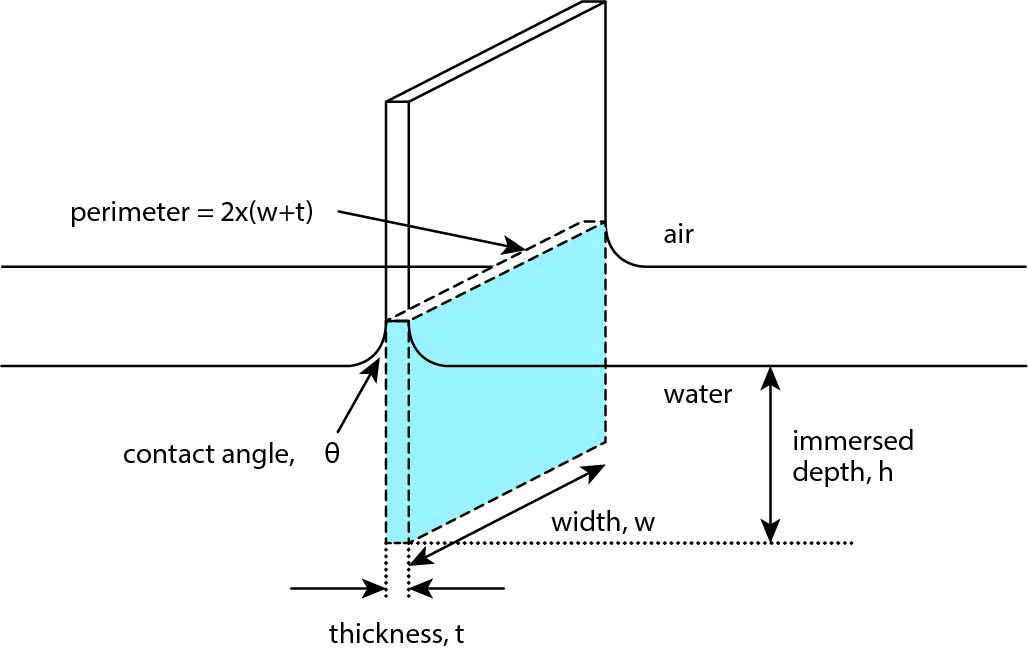The force affecting the Wilhelmy plate can be written as

F= γlPcosθ,

, where γl is the surface tension of the liquid, P is the perimeter of the plate (P=2w + 2t) and θ is the contact angle between the plate and the liquid. As complete wetting is assumed, the contact angle is 0 and Cosθ = 1. The surface tension can then be calculated as

γl = F/P

Wilhelmy plate measurements can also be done in so-called static or continuous mode where Wilhelmy plate remains in contact with the liquid during the entire measurement cycle.

### Platinum rod

Both of the above-mentioned approaches require a relatively large amount of the liquid (typically above 10 milliliters) to be used to ensure complete wetting of the probe. It is possible to use a sample vessel with a smaller diameter to decrease the total volume required. However, there is a limit. When the edges of the sample vessel and the probe are too close to each other, the balance can be affected by the meniscus that forms between the liquid and the edge of the vessel. To avoid this problem, the user must allow enough room between the probe and the sidewall of the sample container, ~ 2 mm or so.

As with any of these techniques, the accuracy of the measurement is affected by the accuracy with which one measures the geometry of the probe. The measurement accuracy of the platinum rod is likely to be less than the du Noüy ring or the Wilhelmy plate which translates to larger errors in the surface /interfacial tension results. Therefore, the platinum rod approach is only recommended when the volume of the sample is limited. The method and calculations are based on the same principle as the Wilhelmy plate method.

## Surface Tension Measurements with Optical Tensiometer

Optical tensiometers are utilized to measure static and semi-dynamic surface tension values. The main components of the optical tensiometer are a camera, dispenser to dispense a drop, sample stage, and the light source to illuminate the drop on the sample stage. Optical tensiometers range from completely manual systems to fully automated instruments.

Pendant drop method

Surface tension measurements can be performed optically using a pendant drop shape analysis. The shape of the drop hanging from a needle is determined from the balance of forces which include the surface tension of the liquid being investigated.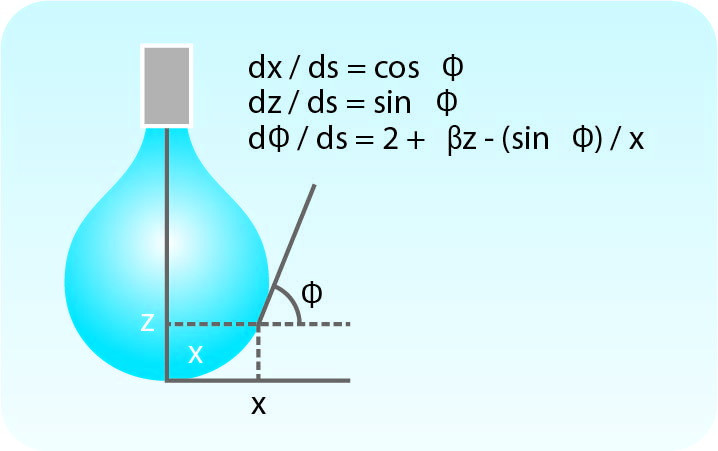The surface or interfacial tension can be related to the drop shape

γ = ΔρgR0

, where γ is the surface tension, Δρ is the density difference between fluids, g is the gravitational constant, R0 is the drop radius of curvature at the apex, and β is the shape factor. β can be defined through the Young-Laplace equation expressed as 3 dimensionless first-order equations.

Modern computational methods using iterative approximations allow for solutions of the Young-Laplace equation to be found. Thus, the surface or interfacial tension between any two immiscible fluids with known densities can be determined. For optical tensiometry the size of the droplet is important and it should have a tear or pendant shape. Care must be taken to ensure that the needle tip is not influencing the drop shape. When measuring surface tension, the density difference between liquid and gas (usually air) is large enough that volumes between 5 μl to 20 μl are generally sufficient to provide pendant or tear shapes. When measuring interfacial tensions, both density difference and interfacial tension influence the required volume to achieve a pendant or tear shape drop. As a guideline, the smaller the density difference, the larger the volume required.

Rising bubble

There is also a possibility to measure surface tension in an inverted measurement. The name of this method is not as well established but it is sometimes called a rising bubble or an inverted pendant drop. The method is quite often used to measure interfacial tension between two immiscible liquids but it can also be utilized for surface tension measurements. In this method, the liquid is placed in a transparent cuvette and a hooked needle is used to create an air bubble. Surface tension is calculated in the same way as with a standard pendant drop experiment. In surface tension measurements, the method is mainly used when very long surface tension measurements need to be done.

## Surface Tension Measurements at Extreme Conditions

Most of the time surface tension measurements are done at ambient conditions but in some cases, temperatures and even pressures need to be controlled.

Temperature control is possible with both optical and force tensiometers. With force tensiometer, the measuring cup can be cooled or heated to reach the desired temperature. Temperature ranges from -20 °C to 200 °C.

With the optical tensiometer, the measurement is done by having the liquid in a cuvette which can be cooled or heated. A rising bubble method is then used to define the surface tension value.

With the optical tensiometer, it is also possible to measure surface tension under pressure. This is important especially in enhanced oil recovery applications where high temperatures and pressures are needed to evaluate phenomena at reservoir conditions. Pressure control is also needed if aqueous solutions need to be heated close to and above 100 °C to prevent bubble formation.

## Standards for Surface Tension Measurements

There are several standards available for surface tension measurements. Most of the standards utilize force tensiometer with either du Noüy ring or the Wilhelmy plate method but there is also a standard for surface tension measurement using the pendant drop method.

## Related products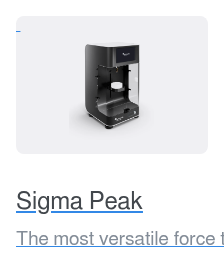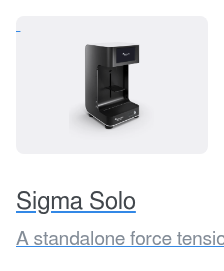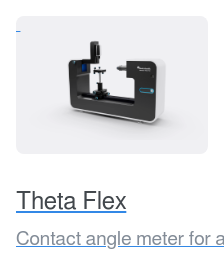#### Save it for later?

We have put all the knowledge of this page in a guide for you to read when you have the time.## Save it for later?

We have put all the knowledge of this page in a guide for you to read when you have the time.